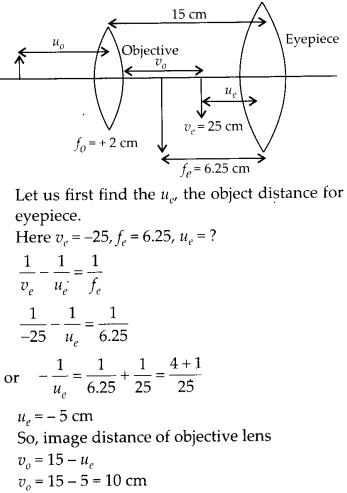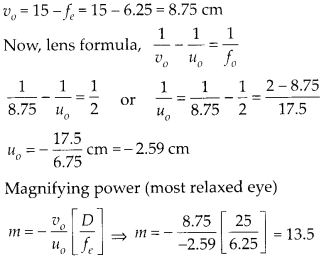Enlightened

# Question 11: NCERT Solutions for 12th Class Physics: Chapter 9-Ray Optics and Optical Instruments

• 0

Question 11: NCERT Solutions for 12th Class Physics: Chapter 9-Ray Optics and Optical Instruments

A compound microscope consists of an objective lens of focal length 2.0 cm and an eyepiece of focal length 6.25 cm separated by a distance of 15 cm. How far from the objective should an object be placed in order to obtain the final image at (a) the least distance of distinct vision (25 cm), and (b) at infinity? What is the magnifying power of the microscope in each case?

Share

1. Solution:
(a) We want the final image at least distance of distinct vision. Let the object in front of objective is at distance υ0.Now we can get required position of object in point of objective.(b) We want the final image at infinity. Let us again assume the object in front of objective at distance υ0.

The object distance υe for the eyepiece should be equal to ƒe = 6.25 cm to obtain final image at ∞. So, image distance of objective lensCheck the complete chapter with solutions.

NCERT Solutions for 12th Class Physics: Chapter 9-Ray Optics and Optical Instruments

• 0